What is AC voltage capacitors?
2022-12-20

When electrons move in an alternating direction in a conductor, an alternating current is produced. Electrons, in electronics, move from a negative potential to a positive potential. If the potentials between two terminals in a fixed time interval are switched, there is a production of alternating current. The difference is expressed in volts between the positive and negative terminals. Therefore, the term used to determine the value of the difference between the potentials of terminals when an alternating current is flowing is AC Voltage.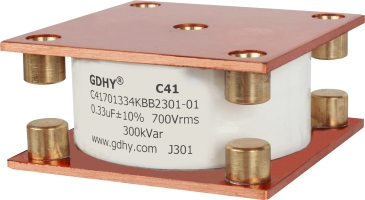The electronic components used in a circuit to store and release electricity are known as AC voltage capacitors and they have the tendency to pass the alternating current without passing the direct current.

Capacitors, when connected across a DC Voltage, get charged and start acting like temporary storage devices. When the capacitors are charged fully no more electrons will flow on its plates. Therefore, once the capacitor gets fully charged, it blocks the DC current.

Now, if the alternating voltage is applied to a capacitor, there will be simultaneous charging and discharging. The rate of the frequency will be determined by the frequency of the supply AC voltage. Therefore, in an AC circuit, the capacitance of the capacitor which is constantly charged or discharged depends on the frequency of the input signal.

This article gives a clear knowledge of electric circuits when one uses an AC voltage across a capacitor. In this circuit layout, we have linked a capacitor and an AC voltage V, represented by the symbol “~.”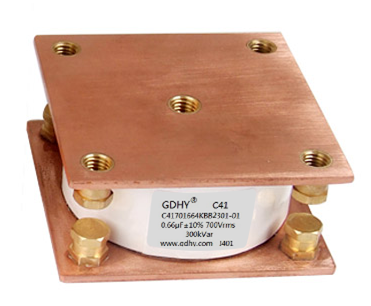The voltage in the circuit produces a potential difference across its terminals that varies sinusoidally.

The expression about the potential difference v, or the AC voltage is given below:

V=Vm sinωt

Where,

Vm = amplitude of the oscillating potential difference

ωt = angular frequency

We can calculate the current that is available in the resistor of the present voltage by using Kirchhoff’s loop rule.

Here is the expression of Kirchhoff’s loop rule:

v(t)=0

### Capacitance in AC Circuit and Capacitive Reactance

We can write an expression for the capacitor:

V =q / C

As mentioned earlier about v, we can rewrite the expression as:

Vm sinωt q / C

We can calculate the amount of current through the circuit by using this relation,

i dq / dt

i = d(vmCsinωt) / dt ωCvmcosωt

i = im sin(ωt + π/2)

In the above expression, a relation is used which is

Cosωt = sin(ωt + π/2)

Also, we can rewrite the amplitude of the current as:

Im = ω Cvm

Or, we can express it as

im = vm / (1/ ωC)

In this expression, 1/ωC can be taken as the equivalent to the resistance of the device.

This is why the term for this expression is said to be capacitive resistance. Xc is the symbol used for the captive resistance.

Xc = 1 / ωC

Also, we can calculate the amplitude of the current in the circuit by using the following relation:

Im = vm / XC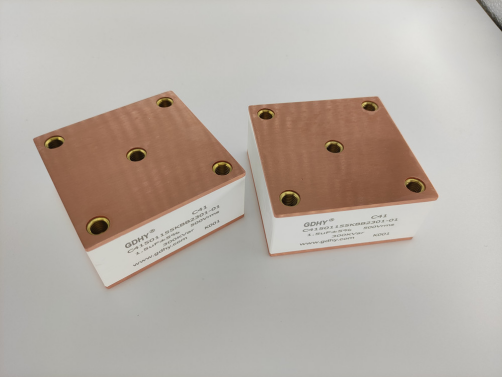### How does a Capacitor work in an AC Circuit?

In an electric circuit, a capacitor puts a direct linkage with the AC supply voltage. When there is an alteration in the supply voltage (voltage increases or decreases), then the capacitor gets charged or discharged by following the change in voltage.

When current passes through the circuit, it will follow one direction, and then in the other direction without letting any actual current to pass through the capacitor.

However, in a DC circuit, the scenario is different. When current flows through a capacitor that is linked with the DC circuit, the capacitor plate possesses both positive and negative charges.

AC capacitors circuits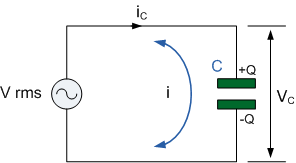When a capacitor is linked with an AC circuit, it will consecutively charge and discharge at a rate calculated by the frequency of the supply. In AC circuits, capacitance varies with frequency as the capacitor is being charged and discharged constantly.

What is the role of capacitor in AC and DC circuit?

Role of a capacitor in DC circuit

In a DC (direct current) circuit, a capacitor gets charged up at a slower rate. A capacitor gets charged up to its supply voltage but opposes the further passage of current through it. It blocks the current flow as the dielectric of a capacitor is non-conductive and an insulator.

Role of a capacitor in AC circuit

When a capacitor is used in an AC circuit, it charges and discharges to change the supply voltage. According to the record, the current becomes directly proportional to the voltage rate at its greatest, across the plates.

The capacitors that are linked in an AC circuit blocks the power supply when they are fully charged. When there is an AC power supply in the circuit, the capacitors will charge and discharge alternatively at a rate determined by the supplied frequency.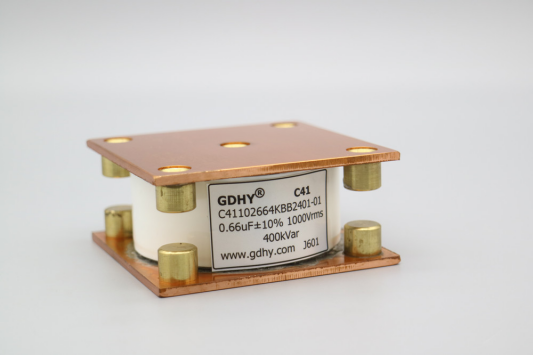Function of capacitor in AC circuit

We know that capacitors are used to store energy on their conductive plates in the form of an electrical charge.

1. Capacitors are used to build up voltage above the input voltage. It helps in the smooth current fluctuations.

2. Most importantly, capacitors are used in rectifier circuits to level the current fluctuations.

3. Capacitors are also used to block the DC static voltage and allow AC signals to pass from one circuit area to another. These types of capacitors are known as coupling capacitors.

4. To eliminate any AC signal at the DC bias point, decoupling capacitors are used.

5. The starting torque can be improved through capacitors. Also, capacitors are good to go in the single phase.

6. Also, capacitors are used to improve the power factor in power systems.

AC through capacitor - derivation

We can name a pair of conductors as a capacitor, separated by some medium. When we link a capacitor with an AC circuit, we can find the current flowing through it.

When we connect a lamp in that circuit, the lamp glows, which shows the current passage in the AC circuit. We concluded that a capacitor is a conductor in the AC circuit, but works as an insulator in the DC circuit.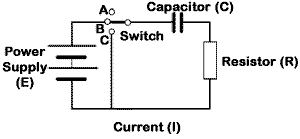Suppose in a circuit the alternating voltage source is V = V0 sinωt and the capacitance of the capacitor is C.

At any time t, the charge on the capacitor is q and the current flowing is i.

As there is no resistance, the instantaneous potential drop in the circuit across the capacitor will be q/c and it must be equal to the applied alternating voltage. Therefore,

q/c =V0 sinωt

The instantaneous current in the circuit is i = d/t, therefore,

i = (dq / dt)(CV0 sinωt)

i = (dq / dt)(CV0 cosωt)

i =( V0 / (1/ωC) ) cosωt

i = i0 cosωt

q / c = i0 sin(ωt+π/2)

where i0 = V0 / (1/ωC)= peak current value

If we compare the peak value of current with V = V0 sinωt, we observe that the current leads the emf in a perfect capacitor by a phase angle of 1/2.

Now, if we compare the peak value of current with ohm’s law, we observe that the quantity 1/ωC possess a dimension of the resistance, therefore

XC = 1/ωC = (1/2)πfC

This is known as capacitive reactance.

From this equation, we observe that if there is an increase in the frequency of the current, the capacitive reactance decreases, and when the frequency is equal to zero the capacitive reactance is infinite for direct current.

RECOMMENDED FOR YOU
GDHY Revolutionizing Capacitor Design to Enhance Performance and Efficiency in Small Welding and Quenching Equipment
Precautions for using film capacitors
Start capacitor in AC Induction Motor
How does metallized polyester film capacitors work in the application?
How to chose water cooled resonant capacitor ?
What is an axial film capacitor?
What is a plastic film capacitor?
IGBT Snubber Capacitors Selection Guide
What is a metallized polyester film capacitor?
What is a coupling capacitor and a bypass capacitor?
Get In Touch With Us
If you contact us now for more details, you can take samples for free. Our service team will get back to you with in 24 hours normally !